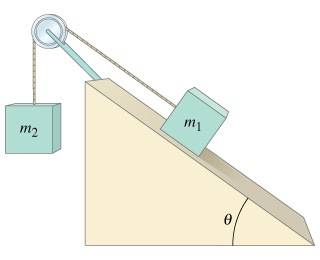# Kinetic Friction with Blocks Need Help.

Larin

## Homework Statement

Suppose the coefficient of kinetic friction between m1 and the plane in the figure is μk=0.20, and that m1=m2=2.2kg.
a) As moves down, determine the magnitude of the acceleration of m1 and m2 given θ=28o.b) What smallest value of μk will keep this system from accelerating?

F=ma

## The Attempt at a Solution

I am not sure even where to begin.